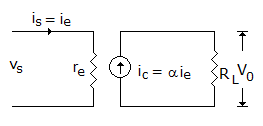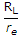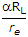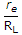# Electronics and Communication Engineering - Analog Electronics

6.

In a transistor leakage current mainly depends on

 A. doping of base B. size of emitter C. rating of transistor D. temperature

Explanation:

No answer description available for this question. Let us discuss.

7.

In a CB amplifier the maximum efficiency could be

 A. 99% B. 85% C. 50% D. 25%

Explanation:

No answer description available for this question. Let us discuss.

8.

Assertion (A): As per Miller theorem a capacitance C from input to output appears as a much larger capacitance across input terminals

Reason (R): In a lag network the output voltage appears across capacitor

 A. Both A and R are correct and R is correct explanation for A B. Both A and R are correct but R is not correct explanation for A C. A is correct R is wrong D. A is wrong R is correct

Explanation:

No answer description available for this question. Let us discuss.

9.

When a transistor is connected in common emitter mode, it with have

 A. negligible input resistance and high output resistance B. high input resistance and low output resistance C. medium input resistance and high output resistance D. low input resistance as well as output resistance

Explanation:

No answer description available for this question. Let us discuss.

10.

In the equivalent circuit of figure voltage gain isA. a B.C.D.